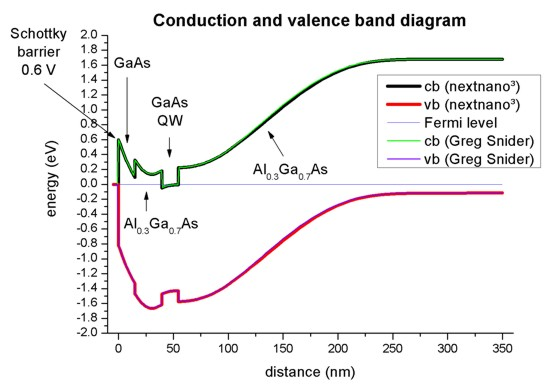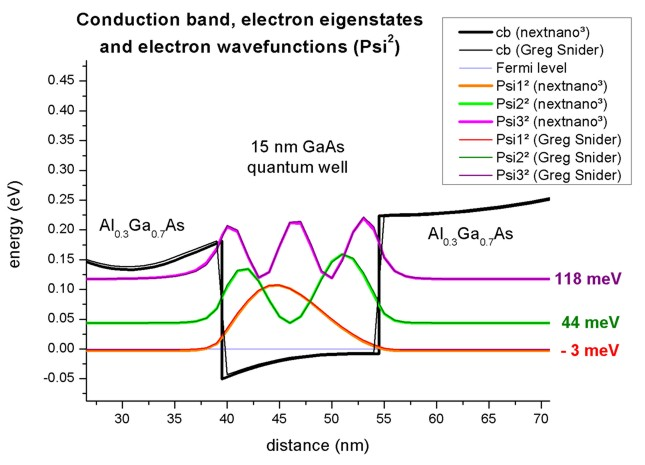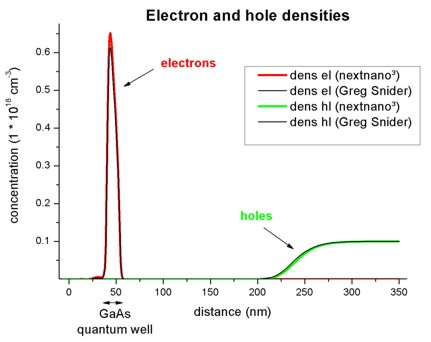nextnano.com  GUI: nextnanomat  Tool: nextnano++  Tool: nextnano³  Tool: nextnano.NEGF  Download | Search | Copyright | News | Publications  * password protected nextnano³ software1D Schrödinger-Poisson

# nextnano3 - Tutorial

## Schrödinger-Poisson - A comparison to the tutorial file of Greg Snider's code

Author: Stefan Birner

`==> `This is the old website: A new version of this tutorial can be found here.

```-> Greg_Snider_MANUAL_1D_nn3.in          / *_nnp.in - ```input file for nextnano³ and nextnano++ software``` -> Greg_Snider_MANUAL_1D_nn3_analytic.in / *_nnp.in - ```input file for nextnano³ and nextnano++ software (analytic doping function)``` -> Greg_Snider_MANUAL_2D_nn3.in          / *_nnp.in - ```input file for nextnano³ and nextnano++ software
```-> Greg_Snider_MANUAL_2D_nn3_analytic.in / *_nnp.in - ```input file for nextnano³ and nextnano++ software (analytic doping function)

We appreciate that Greg Snider (University of Notre Dame) provided his code, the manual and the input files free of charge, so that we could use it here as a test case.
His 1D Poisson/Schrödinger code can be obtained from here: http://www.nd.edu/~gsnider/
This tutorial is based on his manual ('`1D Poisson Manual.pdf`',``` MANUAL.EX```).

## Schrödinger-Poisson - A comparison to the tutorial file of Greg Snider's code

We simulate a structure consisting of

 surface Schottky barrier of 0.6 V 15 nm GaAs n type doped (1018 cm-3) 20 nm Al0.3Ga0.7As n type doped (1018 cm-3) 5 nm Al0.3Ga0.7As 15 nm GaAs quantum well 50 nm Al0.3Ga0.7As 250 nm Al0.3Ga0.7As p type doped (1017 cm-3) substrate

The grid resolution is 1 nm with the exception of the 250 nm layer which has a resolution of 5 nm.
The dopants are assumed to be fully ionized.
The temperature is 300 K.
The Schrödinger equation will be solved between 5 nm and 195 nm.

### Doping profile

We consider two further impurity profiles resulting from ion implantation using LSS theory.
The donor and acceptor profiles are written out to the file "`doping_concentration1D.dat`" and look as follows:The relevant parameters are:

 implant dose [1/cm2] projected range Rp [nm] projected straggle Delta Rp [nm] donor 2 * 1012 86 44 acceptor 1 * 1011 75 20

For further details on the LSS theory (ion implantation) and on the doping profiles, please check the relevant keyword``` \$doping-function```.

### Conduction and valence band edges

The following figure shows the conduction and valence band edges as well as the Fermi level (which is constant and has the value of 0 eV) for the structure specified above. These bands are the solutions of the the self-consistent Schrödinger-Poisson equation.
Both codes, nextnano³ and Greg Snider's "1D Poisson" lead to the same results.### Electron eigenstates and eigenfunctions

Inside the GaAs quantum well there are three confined electron states. The ground state is below the Fermi level and thus occupied.
The following figure shows a zoom of the GaAs Quantum well.The wave functions as calculated with nextnano³ are nearly identical to Greg Snider's "1D Poisson" code, as well as the energies.
However, there are tiny differences which is not too suprising as the conduction band profile is not completely identical:
nextnano³ uses multiple points at material interfaces which lead to sharp (abrupt) interfaces.

nextnano³ interface: 39.5 nm (two grid points, one for GaAs and one for AlGaAs)
54.5 nm (two grid points, one for GaAs and one for AlGaAs)

Greg Snider's interfaces: There is a grid point at 39 nm (AlGaAs) and the next one at 40 nm (GaAs),
and at 54 (GaAs) nm and the next one at 55 nm (AlGaAs).
Thus at 39.5 and 54.5 nm, an interpolated value is used in the plot of the
conduction band diagram.

 Electron states nextnano³ Greg Snider's "1D Poisson" code E1 [meV] `-  3.0` `-  1.3` E2 [meV] `  43.5` `  44.0` E3 [mV] ` 117.5` ` 117.8`

### Electron and hole densities

The electron and hole densities are depicted in this figure. Again, there is also nice agreement between the two codes.The integrated electron density in the GaAs quantum well region is 0.664 * 1012 cm-2. (Greg Snider's result: 0.636 * 1012 cm-2)
The integrated hole density in the right most Al0.3Ga0.7As region is 1.033 * 1012 cm-2. (Greg Snider's result: 1.085 * 1012 cm-2)
The relevant output files are:
```- densities/int_el_dens.dat - densities/int_hl_dens.dat```

This tutorial shows very nicely that both codes, nextnano³ and Greg Snider's "1D Poisson" lead to the same results.
Greg Snider's 1D Poisson/Schrödinger code can be obtained from here: http://www.nd.edu/~gsnider/

### 2D simulations

`-> Greg_Snider_MANUAL_2D_nn3.in / *_nnp.in`

The following figure shows the conduction band profile and the ground state wave function (probability density psi²) of the same structure in a 2D simulation where the y direction has been assumed to be of length 100 nm with periodic boundary conditions.• Please help us to improve our tutorial! Send comments to``` support [at] nextnano.com```.